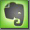Speed of Sound, Sound Waves, Temperature and Humidity

Sound Waves and the Speed of Sound

Sound waves are longitudinal waves (also called compression waves). Vibrating objects excite air molecules that start oscillating. (Actually air molecules oscillate always in some way but with strong excitement the oscillation becomes larger.) The vibration of air molecules takes place in the direction of the excitement, with the next layer of molecules excited by the first layer. The molecules oscillate in the frequency of the initial exciting vibration, creating oscillating of higher and lower density of molecules. Pushing layer by layer forward, a longitudinal wave is created.The wave length of this longitudinal wave is the ratio between the speed of sound and the initial frequency. A higher frequency generates a shorter wavelength and a lower frequency generates a longer wave length. Wave lengths in the audible sound range differ between about 12m (28Hz) and 2,1cm (16kHz).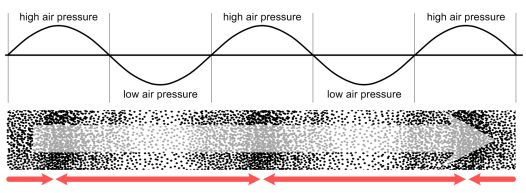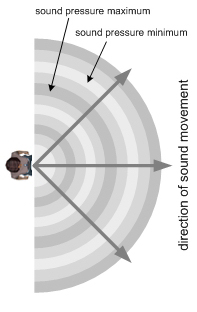propagation of sound as a longitudinal wave  -  oscillation of air molecules   -   relationship of movement and pressure

The speed of sound at 20°Celsius (68°F) is 343 m/s  =  1236 km/h (768 mph)  =  Mach 1.     The speed of sound in water is about 1500 m/s and in steel 5800 m/s. Speed is the measure of travelled distance per time expressed in m/s (meters per second), km/h (kilometers per hour) or mph (miles per hour). The faster something travels the more distance it covers in the same period of time.

Speed of Sound and Distance Calculations

Compared to the speed of light (about 300 000 000 m/s) the speed of sound is "slow". The distance of any event generating light and sound simultaneously can be determined by measuring the difference of the arriving time of both. Compared to the speed of sound the speed of light is so high that the arriving time can be assumed as 'zero'. With the sound signal arriving a certain time later, this time can be re-calculated to identify the distance:

Travelling Distance  =  Speed * Time

For instance a lightning generates light and thunder, both simultaneously. If the sound arrives three seconds after the light, the origin must be about 1km away:
Distance = 343 m/s * 3 seconds = 1029 meter.

Temperature

Temperature is the strongest influence in the speed of sound. The speed of sound depends mostly on the temperature of air and not on air pressure or humidity. Both have only a relative small influence in the speed of sound. With higher temperature the speed of sound will increase. At higher temperatures with higher kinetic energy molecules also have more energy and are able to vibrate faster. The following equation is an estimation that it accurate enough for our purposes.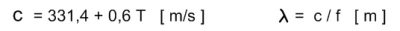Why is the speed of sound important for sound reinforcement business? With the speed of sound the wave length associated with a certain frequency will change! With a higher temperature the speed of sound will be faster and there as the wave length will be longer !

With a changing wave length of a given frequency physical rooms react different. On the other side standing waves in a room (room mode) are fixed by the physical dimension of the room's walls. With higher temperature here the frequency of the room modes will increase!

Humidity

Humidity has a small but measurable effect on sound speed. Compared to the influence of temperature it can be neglected. But humidity has a strong influence of high frequency propagation. The dryer the air is the higher is the damping of high frequencies. At 20% relative humidity a 10kHz tone is damped by over 24dB at 100 meters, but at 80% relative humidity it is damped only by 9 dB per 100 meter.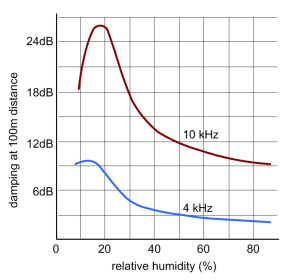Refraction
 Different temperatures cause different speed of sound. 0°C: speed of sound = 331 m/s 15°C: speed of sound = 340 m/s 25°C: speed of sound = 346 m/s With different speed the air has a different refraction index.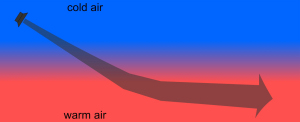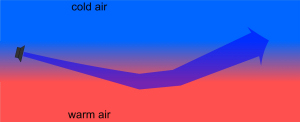sound refraction because of different speed of sound (because of different temperatures)

Influence of Wind

Wind as movement of air particles in the same direction or in the opposite direction as the movement of sound will change the sound direction. The wind speed decreases further to the ground because of frictions with the surface and objects on the surface. As the wind speed is different in these single layers it 'carries' the sound differently.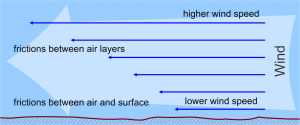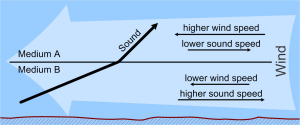different wind speed because of friction refraction of sound because of different wind speeds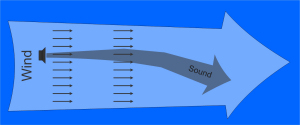wind in the same direction as sound wind in opposite direction as sound